Disclaimer: This is an example of a student written essay.

Any scientific information contained within this essay should not be treated as fact, this content is to be used for educational purposes only and may contain factual inaccuracies or be out of date.

# Changing Output Voltage of DC – DC Converters

 ✅ Paper Type: Free Essay ✅ Subject: Physics ✅ Wordcount: 3599 words ✅ Published: 1st Feb 2018

Ben Harper

The Purpose of this investigation is to look at the effects of changing various components in a 3 volt to 9 volt DC – DC converter will have on the output voltage. The results collected will be compared to theoretical values in order to find out whether or not they match and if not why.

Background

DC – DC converters are very important components in many different circuits, they are used in laptops, cars, and cell phones, mainly devices that receive their power from batteries. There are often sub circuits within complex circuits and these sub circuits often require a specific voltage that is different from the voltage that is flowing through the circuit, this is where the DC – DC converter comes in, it is used to either step up or step down the voltage so that the sub circuit can function properly. One other use is to step up the voltage of a battery that is low on power, when batteries become low on power their output voltage can decrease making the circuit unable to function but with a step up DC – DC converter the lowered voltage can be stepped up and increased enough to allow the circuit to continue functioning for longer. This method is much more efficient than using multiple batteries. Ultimately DC – DC converters play various roles in circuits but they are essential and effective.

Research

Resistors

Resistors are small devices usually made of ceramic that control the amount of current flowing through a circuit. The resistance of resistors are measured in ohms (â„¦) When the resistance of a resistor is high the current is low and when the resistance is low the current is high. Voltage, current and resistance is all connected to one another through Ohm’s law. Ohm’s law can be stated in words as the current in a resistive circuit is directly proportional to its applied voltage and inversely proportional to its resistance (Ffden-2.phys.uaf.edu, 2014). In the form of an equation it can be defined as: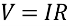Resistors can be found in almost any circuit and play a very important role. Certain components within circuits cannot handle high levels of voltage and will overload and become unusable if too much voltage flows through them, properly placed resistors can stop this from happening. There are many other types of resistors such as variable resistors and foil resistors and potentiometers

Capacitors

Capacitors are very common components that can be found within most circuits and have multiple uses. These components have the ability to store an electrical charge and then discharge at certain intervals of time. They are used for multiple reasons, one of which is their ability to smooth a current. Having a smooth current can prevent false triggering of sensitive components such as relays. Capacitors are measured in capacitance and this is found using the formula: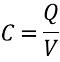A capacitor contains only two conductors that are separated by a single insulating material called a dielectric. The dielectric can be one of many different things, plastic, paper, ceramic or air. The two plates can be made of aluminium or a thin film of metal. Electrolytic capacitors have positive and negative leads, this means that they are polarized, the capacitor must be placed the right way around or else the oxide insulator on one of the foils will erode and cause the component to become conductive causing it to conduct large amounts of current which results in the electrolytic fluid boiling, building up pressure and ultimately popping. Non-electrolytic capacitors tend to have a significantly lower capacitance and are normally used to smooth the current in a circuit.

Electromagnetic induction coils

Electromagnetic induction a process that takes place when a conductor is present in a varying magnetic field or when a conductor is moves through a non-changing magnetic field. These actions cause voltage to be produced across the conductor. This whole process induces an electrical current. The discovery of electromagnetic induction was made by Michael Faraday in 1981 and he is known for the equation named Faraday’s law of electromagnetic induction. The equation is as follows: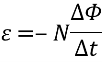Flux is a measurement of how much of a vector field passes perpendicularly through a given area (Hyperphysics.phy-astr.gsu.edu, 2014). A vector field is a vector quantity that can have different values, an example of a vector field is the water molecules in a river, in this example the vector quantity changes depending on its location. The vector field that present in electromagnetic induction is dependent on multiple things such as location and number of coils. In Faradays law of electromagnetic induction, flux which is represented by the Phi symbol is found using: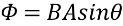The voltage (E.M.F.) created in a coil by a changing magnetic field is proportional to rate in which the magnetic flux changed and the number of winding in the coil (Basic Electronics Tutorials, 2014).

TL499A IC

The TL499A is an integrated circuit (IC) that was created for the purpose of providing adjustable regulated supply voltages, by adding a battery between 1.1 volts and 10 volts, an inductor, a filter capacitor and two resistors the IC can function as a step-up switching regulator. In this specific chip output voltages can vary between 2.9 volts and 30 volts, but this depends on multiple factors such as input voltage, current and resistance. This IC was designed to be a back-up step up switching regulator in the instance of an ac-line failure. The top view of the IC can be seen below in Figure 1.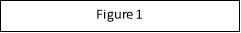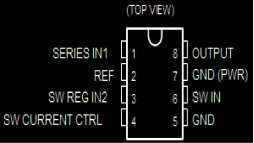Inside of the IC there is a complex circuit that consists of multiple diodes, transistors, resistors and capacitors. Refer to Figure 2 below for a detailed circuit diagram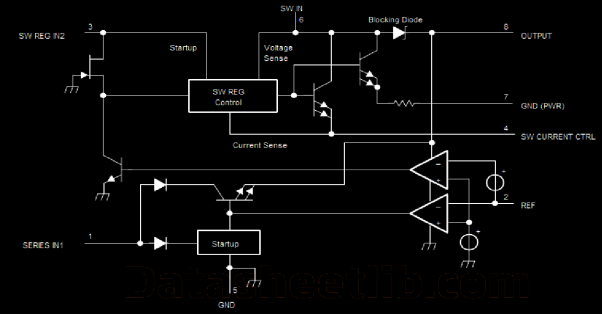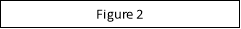Transistors

Transistors are a form of switch, nearly all circuits will have at least one transistor. There are two main types of transistors, the most common is NPN but there is also PNP. When p-type and n-type materials are placed in contact with each other, the junction behaves very differently than either type of material alone. Specifically, current will flow readily in one direction (forward biased) but not in the other (reverse biased), creating the basic diode. This non-reversing behaviour arises from the nature of the charge transport process in the two types of materials. ((Hyperphysics.phy-astr.gsu.edu, 2014)). There are all different kinds of transistors that come in different shapes and sizes but they always have three legs. There is the base, the collector and the emitter. The base is responsible for the activation of the transistor, the collector is the positive leg and the emitter is the negative leg.

Diodes

Diodes are very common components that can be found in many circuits, the function of a diode is to cause electricity to flow in one direction. A diode is an electrical device allowing current to move through it in one direction with far greater ease than in the other. The most common kind of diode in modern circuit design is the semiconductor diode, although other diode technologies exist. (Allaboutcircuits.com, 2014). There are few diodes placed within the DC – DC converter assembled for this investigation but there are multiple diodes found within the TL499A IC.

Hypothesis

By changing resistors R1 and R2 within the Jaycar DC – DC converter kit the output voltage will vary. An increase in the resistance between these two resistors in parallel will result in an increase in output voltage.

Aim

The aim of this experiment is to determine whether or not decreasing the voltage of two parallel resistors will increase the output voltage of the entire circuit. By decreasing the resistance the current will increase and therefore allow a higher output voltage from the IC. “output voltage can be varied from 2.9 V to 30 V by adjusting the input of current which can be changed using the two external resistors” (Datasheetlib.com, 1984).

Experimental procedure

Method

In order to determine the effects that the external resistors, R1 and R2 have on the circuit, they must be replaced with resistors of varying values. Once the resistors have been replaced the circuit must be connected to a power pack and the output voltage must be recorded. This process must be repeated with different resistors until there is a satisfactory amount of data collected.

Materials

• Soldering iron
• Heat proof mats
• Resistors
• Solder
• Sponge

Results

Results: Practical

The second change made was to R2, it was determined after changing the resistor R1 that the current flowing through the circuit was the variable that directly affected the output voltage of the entire circuit. The lower the output current the higher the output voltage was.

 Input Voltage Resistance of R1 Resistance of R2 Output Voltage 3 22000 Ohms 27000 Ohms 4.26 Volts 3 15000 Ohms 180000 Ohms 4.94 Volts 3 33000 Ohms 39000 Ohms 5.83 Volts 3 27000 Ohms 180000 Ohms 7.38 Volts 3 33000 Ohms 220000 Ohms 8.95 Volts 3 47000 Ohms 270000 Ohms 11.92 Volts 3 56000 Ohms 560000 Ohms 14.81 Volts

Results: Theoretical

 Input Voltage Resistance of R1 Resistance of R2 R1, R2 Parallel Output Voltage 3 22000 Ohms 27000 Ohms 12122.45 4.51 3 15000 Ohms 180000 Ohms 13846.15 4.97 3 33000 Ohms 39000 Ohms 17875 6.05 3 27000 Ohms 180000 Ohms 23478.26 7.55 3 33000 Ohms 220000 Ohms 28695.65 8.95 3 47000 Ohms 270000 Ohms 38726.59 11.99 3 56000 Ohms 560000 Ohms 50909.09 14.91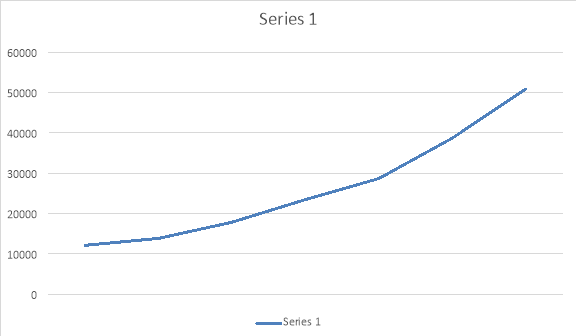The graph above represents the change in resistance as the output voltage increase.

Sources of Error

There are many different possible sources of error within the procedure that is undertaken during this experiment. The power packs at Albany Creek State High school do not all give out the correct amount of voltage; most multimeters are faulty and can give false readings, and with the resistors being constantly soldered and unsoldered in order to replace them there may be a drop in accuracy due to the board melting a small amount or a soldering joint not being done properly. Any other sources of error would be related to physical factors such as temperature and would have minimal and negligible effect on the final results.

Discussion

In any situation where more voltage than what is available is needed when DC current is being used, a step up converter is the solution. The circuit that was put together for this experiment can deliver between 4.5 and 20 volts output depending on the input voltage and the configuration of the resistors. Figure 1 contains a diagram of the TL499A power supply IC. Within the IC there is a series and a switching regulator. The switching regulator is responsible for the operation of the step up pulse width modulated DC – DC converter. A single variable frequency oscillator operates a transistor that is a switch between one side of a boost inductor and ground. In Appendix A it can be seen that the end of the inductor is connected to the positive battery output while the other is connected to pin six of the IC, pin six is the collector of the switching transistor. When the transistor turns on current flowing through L1 rapidly increased while it holds energy within the magnetic field of the inductor. The inductor works by producing voltage when a conductor is moving through a magnetic field and when this field collapses it causes all of the electricity that was being stored in the magnetic field to discharge. When the transistor is powered down it turns off and the magnetic field breaks down producing an immediate increase in voltage. This allows the blocking diode to conduct, therefore transporting the voltage from the inductor to the capacitor that serves as an output filter before it carries on to pin 8 of the TL499a. The second transistor creates a section “of a cycle by cycle current limiting circuit” (Ti.com, 2014). This current turns off the switching transistor when the current through it reaches a predetermined level (Ti.com, 2014). The circuit uses a fixed time arrangement to preserve a controlled output voltage in changing load environments, the changing frequency can be as small as a couple kHz but with the highest load and lowest possible input voltage, it rises to over 20 kHz. DC voltage applied to pin 1 flows into the output at pin 8 through the transistor. “The base of Q3 is driven by an error amplifier, which compares a 1.26 volt reference voltage on its non-inverting input with the voltage at pin 2”. (Datasheetlib.com, 1984) The output voltage is determined by the equation: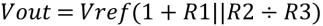By choosing appropriate values for R1 and R2, the output voltage of the entire circuit can be set for any voltage between 4.5 volts and 20. From the data collected it is clear that they the resistance does increase with resistance of R1 and R2. The data does not completely match the theoretical values and the difference between them varies significantly, this is most likely due to the fact that different power packs were used for some of the of the measurements, it could also be an effect of repeatedly soldering and unsoldering the join where the resisters were placed. The clear increase in voltage with the increase in resistance makes it obvious that the results are supportive of the hypothesis and create a clear pattern that shows the increase of voltage that is relative to the resistance. The results do not go past an output voltage of fifteen therefore the limits have not been tested, it has been stated “the TL499A has a minimum output of 2.9 and a maximum output of 30 volts” (Datasheetlib.com, 1984). One possible way of improving the results and increasing the accuracy would be to find an accurate power pack to use on the circuit, another viable option would be to use the same power pack and multimeter for every measurement that is made therefore the results would not be accurate but they would be precise. In order to get accurate results the power pack and multi meter must be tested and it must be made sure that the power pack has the correct output voltage and the multimeter reads the voltage output correctly. Another investigation that this experiment could lead to could be the effect of varying the input voltage to an induction coil.

Conclusion

In conclusion the results collected from the DC – DC converter ultimately support the hypothesis presented earlier in the report that by changing resistors R1 and R2 within the Jaycar DC – DC converter kit the output voltage will vary. An increase in the resistance between these two resistors in parallel will result in an increase in output voltage. The results corroborate with multiple other theories and laws. This DC – DC converter can be altered by simply changing the resistance of resistors R1 and R2 and this is supported by a suitable amount of both primary and secondary data.

Bibliography

Basic Electronics Tutorials. 2014. Electromagnetic Induction and Faradays Law. [online] Available at: http://www.electronics-tutorials.ws/electromagnetism/electromagnetic-induction.html [Accessed: 26 May 2014].

CDS linear, (2014). Linear technology. [online] Available at: http://cds.linear.com/docs/en/datasheet/1111fd.pdf [Accessed 10 Jun. 2014].

Datasheetlib.com, (1984). TL499A Datasheet | DatasheetLib.com. [online] Available at: http://www.datasheetlib.com/datasheet/68927/tl499a_ti-texas-instruments.html [Accessed 10 Jun. 2014].

Ffden-2.phys.uaf.edu. 2014. Ohm’s law. [online] Available at: http://ffden-2.phys.uaf.edu/211.fall2000.web.projects/Jeremie Smith/page4.htm [Accessed: 26 May 2014].

Hyperphysics.phy-astr.gsu.edu. 2014. Magnetic Flux. [online] Available at: http://hyperphysics.phy-astr.gsu.edu/hbase/magnetic/fluxmg.html [Accessed: 26 May 2014].

Jones, A. (2014). How Electromagnetic Induction Creates Current. [online] Available at: http://physics.about.com/od/physicsetoh/g/induction.htm [Accessed 10 Jun. 2014].

Ndt-ed.org, (2014). Electromagnetic Induction. [online] Available at: http://www.ndt-ed.org/EducationResources/HighSchool/Electricity/electroinduction.htm [Accessed 10 Jun. 2014].

People.sinclair.edu, (n.d.). EET 155 Unit 2: Capacitors and Inductors in DC Circuits. [online] Available at: http://people.sinclair.edu/nickreeder/eet155/mod02.htm [Accessed 10 Jun. 2014].

Ti.com, (2014). TL499A | Step-Up (Boost) Converter | Converter (Integrated Switch) | Description & parametrics. [online] Available at: http://www.ti.com/product/tl499a.

View all

## DMCA / Removal Request

If you are the original writer of this essay and no longer wish to have your work published on UKEssays.com then please:

Related Services

Prices from

BHD57

Approximate costs for:

• 1000 words
• 7 day deliveryHumanity University

Dedicated to your worth and value as a human being!

Related Lectures